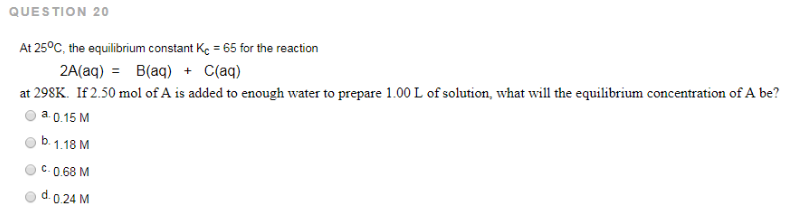# Problem: At 25 °C, the equilibrium constant Kc = 65 for the reaction 2A (aq) = B (aq) + C (aq) at 298K. If 2.50 mol of A is added to enough water to prepare 1.00 L of solution, what will the equilibrium concentration of A be? a. 0.15 M b. 1.18 M c. 0.68 M d. 0.24 M

###### FREE Expert Solution
91% (55 ratings)###### Problem Details

At 25 °C, the equilibrium constant Kc = 65 for the reaction

2A (aq) = B (aq) + C (aq) at 298K.

If 2.50 mol of A is added to enough water to prepare 1.00 L of solution, what will the equilibrium concentration of A be?

a. 0.15 M

b. 1.18 M

c. 0.68 M

d. 0.24 M